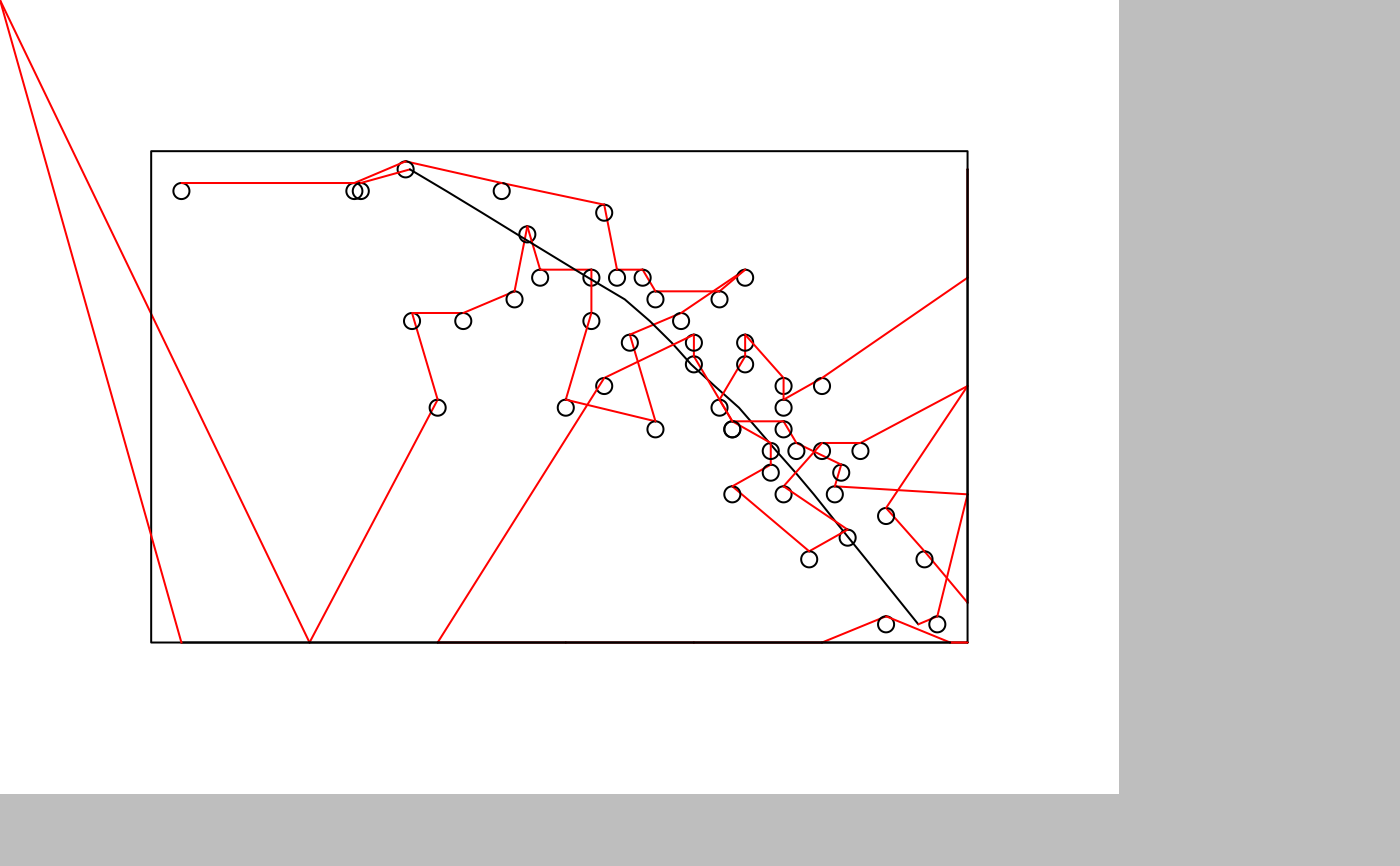axi_dev() opens up a graphic device that takes plotting instructions from e.g. plot() or ggplot2, and renders it with the AxiDraw. ghost_dev() behaves like axi_dev(), but instead of sending instructions to the plotter it will collect them and allow you to preview the movement of the pen and send the instructions to the plotter at a later stage. For more complex plots it is adviced to use the asynchronous ghost_dev() as it makes it easier to pause and rewind the plot if something goes wrong or a pen runs dry.

axi_dev(
paper_size = "A4",
portrait = TRUE,
margins = 20,
tip_size = 1,
color = "black",
ignore_color = TRUE,
ignore_lwd = FALSE,
line_overlap = 0.1,
min_overlap = -20,
draw_fill = TRUE,
hatch_angle = 45,
optimize_order = "all",
pens = list(),
options = axi_options()
)

ghost_dev(
paper_size = "A4",
portrait = TRUE,
margins = 20,
tip_size = 1,
color = "black",
ignore_color = TRUE,
ignore_lwd = FALSE,
line_overlap = 0.1,
min_overlap = -20,
draw_fill = TRUE,
hatch_angle = 45,
optimize_order = "all",
pens = list(),
options = axi_options()
)

## Arguments

paper_size The size of the paper to draw on, either as a numeric vector giving dimensions in mm, or as a standard paper size name. Logical. Is the paper oriented as portrait (i.e. width < height). Will rearrange given paper dimensions to fit. The margins of the paper, in mm. The spec follows that of css, meaning that if it is given as a single value it defines all margins, if given as two values it defines top/bottom, left/right, if it is given as three values it defines top, left/right, bottom, and if it is given as four values it defines top, right, bottom, left. The size of the tip (i.e. the width of the line it draws) of the initial pen The color of the initial pen Logical. Should changes in color output be ignored? If TRUE the plotter will not pause for pen change when the color changes Logical. Should the device ignore the lwd and also just draw lines as a single pen stroke? The overlap between adjacent pen strokes when filling out shapes and drawing thick lines, in mm. Setting this to a negative amount will cause gaps between the lines. If NA the overlap will be calculated from the color/fill alpha, scaled between min_overlap and 0.1. The lower bound in mm of the overlap if it is being calculated from the color/fill alpha. Should be a negative value to ensure low alpha results in gapped hatching. Logical. Should fill be drawn using hatching? Angle in degrees that the hatching of fill should be drawn with. If NA a random angle will be chosen for each fill. Logical. Should the drawing order be optimised so that pen movement is minimised? Will only affect consecutive calls to the same drawing primitive. One or more pen specifications created using pen(). An axi_options object. See the documentation for axi_options() for all the settings.

## Details

At the moment the device does not support text. This will hopefully change in the future.

## Examples

gd <- ghost_dev('A6')
par(mar = c(0, 0, 0, 0))
plot(cars)
lines(lowess(cars))
invisible(dev.off())

gd\$preview(plot_air = TRUE)